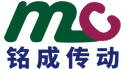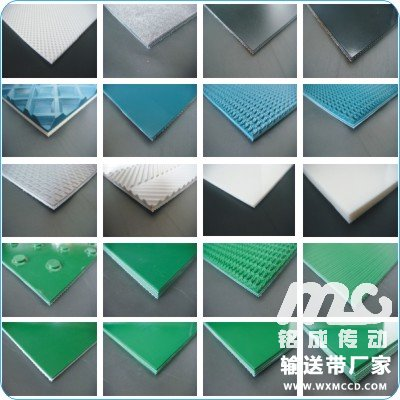#### 推荐产品

1/1162

# 输送带型号,具体有哪些规格品种？

`请教一下输送带规格型号问题，越详细越好`2018-01-06 提问

## 企业回答 本回答由提问者推荐2018-01-06 16:12 回答
```首先输送带是一个很大的范畴，大类可以分为两类，轻型输送带和重型输送带【点击查看更多】轻型输送带型号

□ 分层式织物芯输送带 □ 波状挡边输送带 □ 花纹输送带 □ 环形输送带 □ 尼龙(NN)帆布输送带□ 耐热输送带 □ 耐高温输送带

EP聚酯 :EP100 EP125 EP150 EP200 EP250 EP300 EP350 EP400

B500*3（1.5+1.5）500*3（2+1） 500*3（3+1.5）500*4（3+1.5）500*4（4+2）500*4（4.5+1.5） B500*5（3+1.5） 500*5（4.5+1.5）500*5（4+2） 500*5（6+1.5） 500*5（6+2） 500*6（3+1.5） 500*6（4.5+1.5）等
B500*6（4+2） 500*7 500*8 500*9 500*10 等
B550*3（3+1.5） 550*4（3+1.5）550*4（4.5+1.5）550*4（4+2.）550*5(3+1.5) 550*5(4.5+1.5) B550*5(4+2) 550*6（3+1.5）550*6(4.5+1.5) 550*6（4+2） 550*7 550*8 550*9 550*10等
B600*4（3+1.5）600*4（4.5+1.5）600*4（4+2）600*5（3+1.5） 600*5（4.5+1.5） B600*5（4+2）等
B600*5（6+2） 600*6（3+1.5）600*6（4.5+1.5）600*6（4+2）600*6（6+2） B650*4（3+1.5）650*4（4.5+1.5）650*4（4+2）650*5（3+1.5）650*5（4.5+1.5） 650*5（4+2） B650*5（6+2）650*5（6+3）650*5（6+1.5）650*6（3+1.5）650*6（4.5+1.5）650*6（4+2） B700*5（3+1.5）700*5（4.5+1.5））700*5（4+2）700*6（3+1.5）700*6（4.5+1.5）700*6（4+2） B750*5(3+1.5) 750*5(4+2) 750*5(4.5+1.5) 750*6(3+1.5) 750*6(4.5+1.5) 750*6(4+2) B800*4(3+1.5) 800*4(4.5+1.5) 800*4(4+2) 800*5(3+1.5) 800*5(4.5+1.5) 800*5(4+2) B800*5(6+2) 800*5(6+3) 800*6(3+1.5) 800*6(3+1.5) 800*6(4.5+1.5) 800*6(4+2) B800*6(6+2) 800*6(6+3) 800*7(3+1.5) 800*7(4.5+1.5) 800*8 等
B1000*4（3+1.5）1000*4（4.5+1.5）1000*4（4+2）1000*5（3+1.5）1000*5（4.5+1.5） B1000*5（4+2）1000*5（6+2）1000*5（6+3） 1000*6（3+1.5） 1000*6（8+2）1000*6（3+1.5） B1000*6（4.5+1.5）1000*6（4+2）1000*6（6+2）1000*6（6+3）1000*7（3+1.5） B1000*7（4.5+1.5）1000*7（4+2）1000*7（6+2）1000*7（6+3）1000*7（8+2） B1000*8（3+1.5）B1000*8（4.5+1.5）1000*8（4+2）1000*8（6+2）1000*8（6+3） B1000*8（8+2）等
B1100*4 1100*5 1100*6 1100*7 1100*8等 B1200*4（3+1.5）1200*4（4.5+1.5）1200*4（4+2）1200*5（3+1.5）1200*5（4.5+1.5） B1200*5（4+2）1200*5（6+2）1200*5（6+3） 1200*6（3+1.5）1200*6（3+1.5） B1200*6（4.5+1.5）1200*6（4+2）1200*6（6+2）1200*6（6+3）1200*7（3+1.5） B1200*7（4.5+1.5）1200*7（4+2）1200*7（6+2）1200*7（6+3）1200*7（8+2） B1200*8（3+1.5）B1200*8（4.5+1.5）1200*8（4+2）1200*8（6+2）1200*8（6+3） B1200*8（8+2）等
B1300*4（3+1.5）1300*4（4.5+1.5）1300*4（4+2）1300*5（3+1.5）1300*5（4.5+1.5） B1300*5（4+2）1300*5（6+2）1300*5（6+3） 1300*6（3+1.5） 1300*6（8+2）1300*6（3+1.5） B1300*6（4.5+1.5）1300*6（4+2）1300*6（6+2）1300*6（6+3）1300*7（3+1.5） B1300*7（4.5+1.5）1300*7（4+2）1300*7（6+2）1300*7（6+3）1300*7（8+2） B1300*8（3+1.5）B1300*8（4.5+1.5）1300*8（4+2）1300*8（6+2）1300*8（6+3） B1300*8（8+2）等
B1400*4（3+1.5）1400*4（4.5+1.5）1400*4（4+2）1400*5（3+1.5）1400*5（4.5+1.5） B1400*5（4+2）1400*5（6+2）1400*5（6+3） 1400*6（3+1.5） 1400*6（8+2）1400*6（3+1.5） B1400*6（4.5+1.5）1400*6（4+2）1400*6（6+2）1400*6（6+3）1400*7（3+1.5） B1400*7（4.5+1.5）1400*7（4+2）1400*7（6+2）1400*7（6+3）1400*7（8+2） B1400*8（3+1.5）B1400*8（4.5+1.5）1400*8（4+2）1400*8（6+2）1400*8（6+3） B1400*8（8+2）等
B1500*4（3+1.5）1500*4（4.5+1.5）1500*4（4+2）1500*5（3+1.5）1500*5（4.5+1.5） B1500*5（4+2）1500*5（6+2）1500*5（6+3） 1500*6（3+1.5） 1500*6（8+2）1500*6（3+1.5） B1500*6（4.5+1.5）1500*6（4+2）1500*6（6+2）1500*6（6+3）1500*7（3+1.5） B1500*7（4.5+1.5）1500*7（4+2）1500*7（6+2）1500*7（6+3）1500*7（8+2） B1500*8（3+1.5）B1500*8（4.5+1.5）1500*8（4+2）1500*8（6+2）1500*8（6+3） B1500*8（8+2）等
B1600*4（3+1.5）1600*4（4.5+1.5）1600*4（4+2）1600*5（3+1.5）1600*5（4.5+1.5） B1600*5（4+2）1600*5（6+2）1600*5（6+3） 1300*6（3+1.5） 1600*6（8+2）1600*6（3+1.5） B1600*6（4.5+1.5）1600*6（4+2）1600*6（6+2）1600*6（6+3）1600*7（3+1.5） B1600*7（4.5+1.5）1600*7（4+2）1600*7（6+2）1600*7（6+3）1600*7（8+2） B1600*8（3+1.5）B1600*8（4.5+1.5）1600*8（4+2）1600*8（6+2）1600*8（6+3） B1600*8（8+2）等```